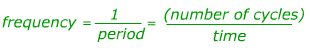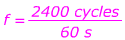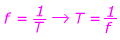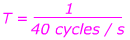Frequency Formula

Frequency is the number of cycles in a unit of time. The “cycles” can be movements of anything with periodic motion, like a spring, a pendulum, something spinning, or a wave. Frequency is equal to 1 divided by the period, which is the time required for one cycle.

The derived SI unit for frequency is hertz, named after Heinrich Rudolf Hertz (symbol hz). One hz is one cycle per second.f = frequency, the cycles in a unit of time

T = period, the time required for one cycle

N = a number of cycles

t = an amount of time

Frequency Formula Questions:

1) A long pendulum takes 5.00 s to complete one back-and-forth cycle. What is the frequency of the pendulum’s motion?

Answer: The pendulum takes 5.00 s to complete one cycle, so this is its period, T. The frequency can be found using the equation:f = 0.20 cycles/s

The frequency of the pendulum is 0.20 cycles/s. The units cycles/s are often written as “Hertz”, with the symbol “Hz”. So, the frequency of this pendulum can also be stated as 0.20 Hz.

2) The tachometer in a car measures the revolutions per minute of the tires (revolutions and cycles are the same thing). A car is travelling at a constant speed, and the tachometer reads 2400 revolutions per minute. What is the frequency of the tires spinning, measured in cycles per second? What is the period, in seconds?

Answer: The number of cycles (revolutions) to consider is 2400. This is the number of cycles that happen in one minute, which is equal to 60 seconds. So, the frequency can be found using the equation:f = 40 cycles/s

The frequency of the tires spinning is 40 cycles/s, which can also be written as 40 Hz. To find the period from this, rearrange the equation that relates period and frequency:T = 0.025 s

The period of the tires spinning is 0.025 seconds.